# The Discrete Fourier Transform

## Steve Mann

Here is a graphical interpretation of the Fast Fourier Transform (FFT).

Let us first begin by understanding the DFT (Discrete Fourier Transform), of which the FFT is a fast (computationally efficient) implementation. The DFT provides a comparison (correlation, inner product) of an arbitrary input signal with a complex exponentials (cosines and sines) at various frequencies. Here, for example, we see a cosine wave sampled at various frequencies:

Sampling of cosines: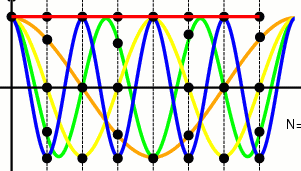(click to see the various cosines sampled).

Fractional frequency is a circular concept, yet when we sample a cosine at a fractional frequency of +5/8 (e.g. 8 samples per second and 5 cycles per second), we get the same result as sampling at +3/8. We'd really like to be able to know the difference between positive and negative frequencies, so that, for example, +5/8 is equialent to -3/8. To do this, we use complex signals, where the real part is a cosine wave, and the imaginary part is a sine wave. Thus, for example, a fractional frequency of +5/8 aliases to a fractional frequency of -3/8. In this way, frequency is a circular concept, as it should be.

Thus there are, for example, eight unique frequencies in a signal that has eight samples. We can also "fftshift" these eight frequency components so that they are ordered from negative to positive frequency:

Reordering into negative frequencies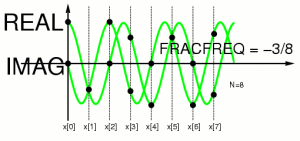Similarly, if we desire, we can also shift the time origin to the center (e.g. in Octave or Matlab, we can use the fftshift() function in both time and frequency):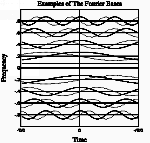If we take enough of these sines and cosines, along rows of a large matrix, e.g. for a 1024 point DFT, the cosines will make a 1024 by 1024 matrix, and so will the sines. The central portion of a Fourier transform matrix looks like this: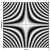(Real part)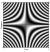(Imag. part)

The Fourier Transform can be computed as a matrix multiplication: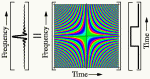The 8 point DFT as a matrix multiplication: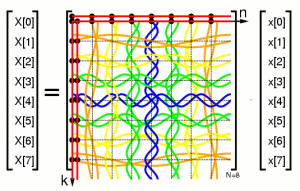To understand how the DFT can be more efficiently computed, let us begin by separating this matrix multiplication into a sum of two matrix multiplications, one for even columns, and one for odd columns:

Separation into even and odd columns: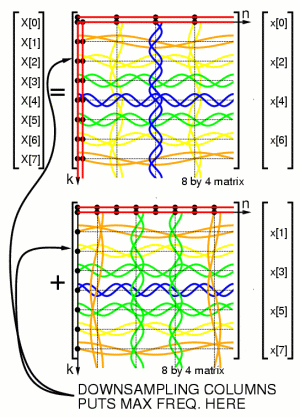Separation into even and even columns: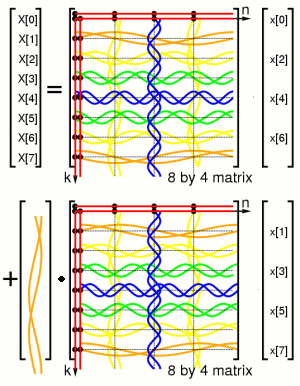where the dot represents element by element (Hadamard) multiplication (as would be denoted by ".*" if this were done in Octave or Matlab); the dot will be omitted in the rest of this document, for simplicity, where it is understood by dimensional consistency where it is assumed.